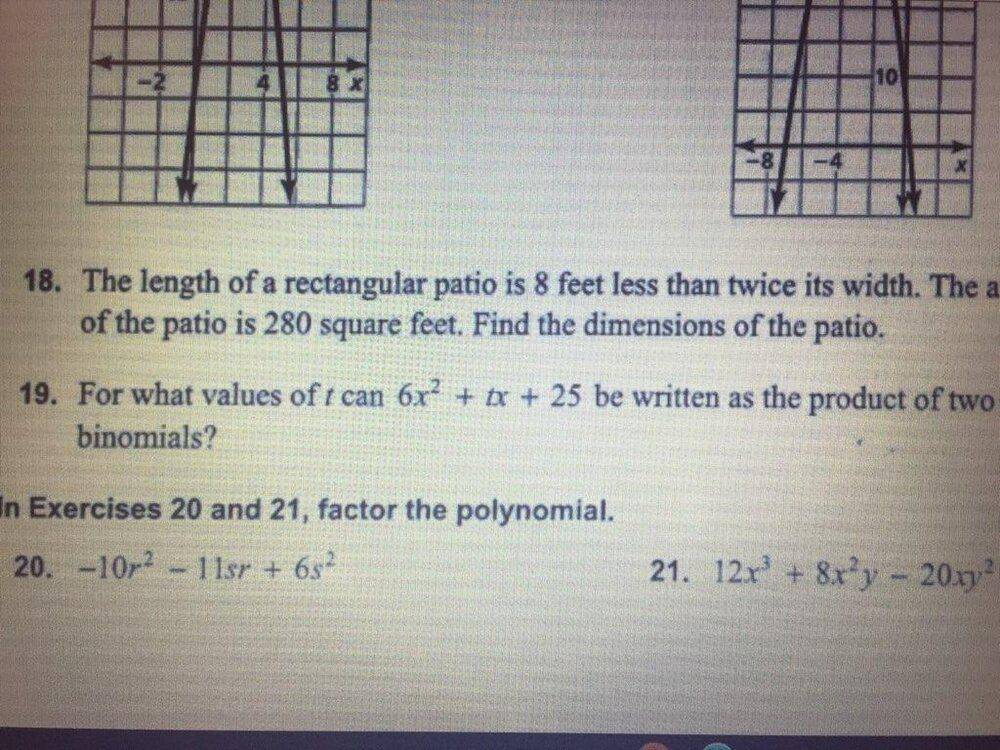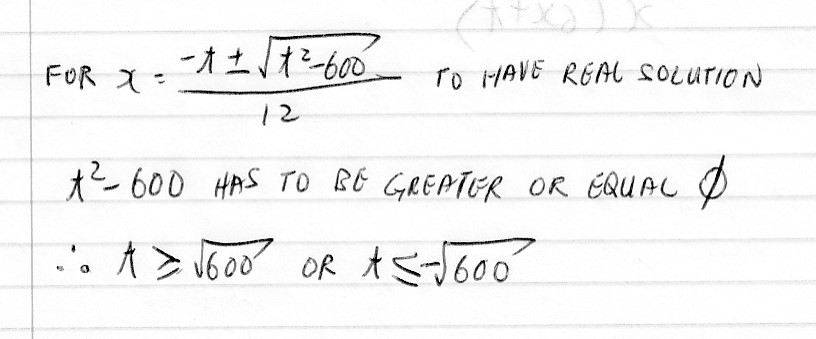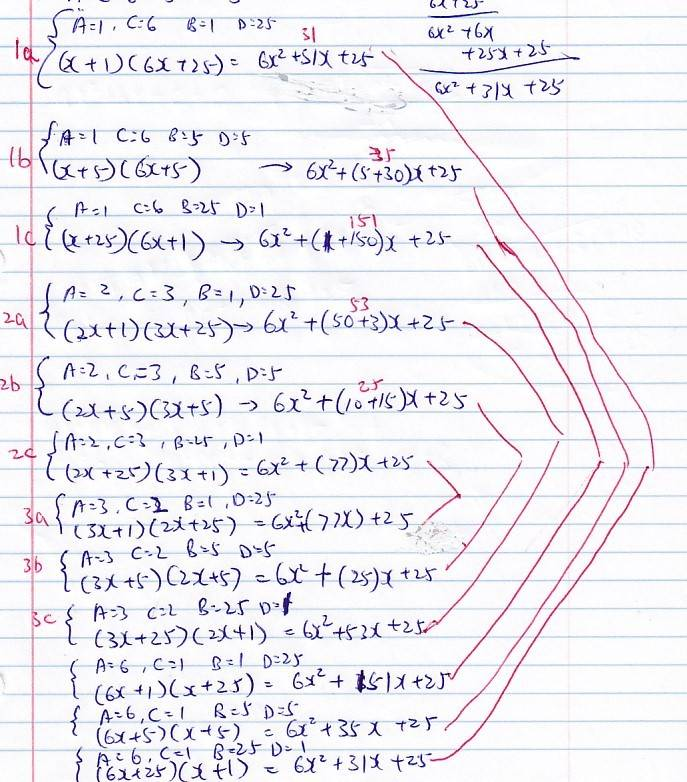# How to solve this polynomial problem

yungman
I have problem solving this. The question is #19 in the first attachment. My work is in the second attachmentI work to this point and get stuck:
https://www.physicsforums.com/attachments/270843

Can anyone help me?

Thanks

Mentor
How many roots does a parabola have if it can be written as product of two binomials? How many does it have if it cannot?

yungman
How many roots does a parabola have if it can be written as product of two binomials? How many does it have if it cannot?
I really don't know, this is a question from my grand daughter and this caught me cold. I am ashamed!

I set the equation to zero to get two roots.

Mentor
In the Quadratic Formula, if the discriminant (the expression under the radical, ##b^2 - 4ac##) is positive, there will be two distinct roots. If the discriminant equals zero, there is one repeated root. If the discriminant is negative, there are no real roots (two complex roots).

Staff Emeritus
Homework Helper
Gold Member
I have problem solving this. The question is #19 in the first attachment. My work is in the second attachment
View attachment 270842

I work to this point and get stuck:
https://www.physicsforums.com/attachments/270843

Can anyone help me?

Thanks
This appears to be from a set of exercises for a lesson on factoring.

There are several methods for factoring a trinomial such as this. What method or methods have your granddaughter studied?

yungman
This appears to be from a set of exercises for a lesson on factoring.

There are several methods for factoring a trinomial such as this. What method or methods have your granddaughter studied?
I have to get hold of her. thanks

I made a mistake on the denominators in my first post, it should be 12 instead of 2. I tried to edit that, but it asked to put in the relevant formula. I can't find how to write formula here. I used to be able to write formula so I don't have to write on paper and scan it in. Seems like it changed since 10 years ago when I was active here.

yungman
Can anyone give me a hint how to look at it? I talked to her, she have not even learn the b^2-4ac stuffs. I don't know why she's even given this problem.

I am really not interested in this, I am drowning on my C++ programming already, just want to resolve this and help her on this one. I haven't even have a chance to work on my C++ today yet!

Thanks

yungman
I have been looking at the question, the only values of t to get 2 binomials is this:Is this right?

Mentor
Right.

Staff Emeritus
Homework Helper
Gold Member
I have to get hold of her. thanks

I made a mistake on the denominators in my first post, it should be 12 instead of 2. I tried to edit that, but it asked to put in the relevant formula. I can't find how to write formula here. I used to be able to write formula so I don't have to write on paper and scan it in. Seems like it changed since 10 years ago when I was active here.
I suppose that you are referring to the quadratic formula:
The solution to the general quadratic equation: ##ax^2+bx+c=0 ##
is given by ##x = \dfrac{ -b \pm \sqrt{b^2-4ac} }{2a} ##

So, yes, you left the factor of ##a## out of the denominator. In this case ##a=6##.

In addition to what @Mark44 said, if ##b^2-4ac## is a perfect square, then ##ax^2+bx+c ## can be written as the product of two binomials. For your (granddaughter's) problem, you need ##t^2-600## to be a perfect square, (as well as being positive, of course).
This problem may be done this way, but it will be quite cumbersome. There are at least 6 possible values for t.

Another way to do this is to systematically find all of the pairs of binomials product give ##6x^2## for the leading term and ##25## for the constant term.

For example: ##(2x + ?)\times(3x + ?)## gives ##6x^2 + ... ##

and ##(?x + 1)\times(?x + 25)## gives ## ?x^2 +?x + 25##

Mentor
@SammyS: Why would you want it to be a perfect square? No one required rational roots or any other special requirement.

yungman
I suppose that you are referring to the quadratic formula:
The solution to the general quadratic equation: ##ax^2+bx+c=0 ##
is given by ##x = \dfrac{ -b \pm \sqrt{b^2-4ac} }{2a} ##

So, yes, you left the factor of ##a## out of the denominator. In this case ##a=6##.

In addition to what @Mark44 said, if ##b^2-4ac## is a perfect square, then ##ax^2+bx+c ## can be written as the product of two binomials. For your (granddaughter's) problem, you need ##t^2-600## to be a perfect square, (as well as being positive, of course).
This problem may be done this way, but it will be quite cumbersome. There are at least 6 possible values for t.

Another way to do this is to systematically find all of the pairs of binomials product give ##6x^2## for the leading term and ##25## for the constant term.

For example: ##(2x + ?)\times(3x + ?)## gives ##6x^2 + ... ##

and ##(?x + 1)\times(?x + 25)## gives ## ?x^2 +?x + 25##
I use your logic to have this, but I have 4 variables A, B, C and D, and I cannot solve it.
##(Ax+B)(Cx+D)=6x^2 + tx + 25##
It would be nice to do this instead of using

##x = \dfrac{ -b \pm \sqrt{b^2-4ac} }{2a} ##

She have not learn this formula, it would be nice to have a way to do this without

##x = \dfrac{ -b \pm \sqrt{b^2-4ac} }{2a} ##

Last edited:
Staff Emeritus
Homework Helper
Gold Member
@SammyS: Why would you want it to be a perfect square? No one required rational roots or any other special requirement.
Looking at the other exercises in the image in the OP, I conclude that these exercises have to do with factoring trinomials into the product of binomials, all with integer coefficients.

Staff Emeritus
Homework Helper
Gold Member
I use your logic to have this, but I have 4 variables A, B, C and D, and I cannot solve it.
##(Ax+B)(Cx+D)=6x^2 + tx + 25##
It would be nice to do this instead of using

##x = \dfrac{ -b \pm \sqrt{b^2-4ac} }{2a} ##
Correct. You have ##(Ax+B)(Cx+D)=6x^2 + tx + 25##.

But A and C are related: ##A\cdot C = 6##. Also ##B\cdot D = 25##.

Now get systematic.
With A = 1 and C = 6, let B = 1 and D =25, and multiply ##(x+1)(6x+25) ## to get ##t##.

Then let B = 5 and D =5, and multiply ##(x+5)(6x+5) ## to get another value for ##t##.

Then let B = 25 and D = 1 ... . Don't forget, B and D can both be negative.

Now consider A = 2 and C = 3. Run through the cases for B and D again.

When can you stop?

yungman
Correct. You have ##(Ax+B)(Cx+D)=6x^2 + tx + 25##.

But A and C are related: ##A\cdot C = 6##. Also ##B\cdot D = 25##.

Now get systematic.
With A = 1 and C = 6, let B = 1 and D =25, and multiply ##(x+1)(6x+25) ## to get ##t##.

Then let B = 5 and D =5, and multiply ##(x+5)(6x+5) ## to get another value for ##t##.

Then let B = 25 and D = 1 ... . Don't forget, B and D can both be negative.

Now consider A = 2 and C = 3. Run through the cases for B and D again.

When can you stop?
Ok, I expanded these out:I see the relation, I connected the pairs with same value of t. These are all the combination. But what does these means?

Does this means t = 25, 31, 35, 53, 77 and 151 all work?

Last edited:
Staff Emeritus
Homework Helper
Gold Member
Ok, I expanded these out:
View attachment 270852

I see the relation, I connected the pairs with same value of t. These are all the combination. But what does these means?

Does this means t = 25, 31, 35, 77 and 151 all work?
Yes. Also 53 .

Additionally, t can be the negative of each of these.

•yungman
yungman
Yes. Also 53 .

Additionally, t can be the negative of each of these.
Thanks, I missed the 53 when I read my notes. It's there also. I put it back in the last post already.

Thanks

Last edited:
Staff Emeritus
Homework Helper
Gold Member
Just to be clear, you can also have binomial products such as ##(3x-1)(2x-25)##, which gives ##t=-77##.

Mentor
Looking at the other exercises in the image in the OP, I conclude that these exercises have to do with factoring trinomials into the product of binomials, all with integer coefficients.
I don't share that interpretation.

yungman
Thanks for the help.

Actually after sitting on it for a day, I really feel the answer in my post #8 is more accurate. I understand the answer in post #15 looks better with integer coef for x.

BUT, this is NOT the full set of answer. Remember, coef for both x and 25 can be fraction, the requirement is just to have the final coef of x to be 6 and final number is 25. Also, coef of x in the two binomial can be of different polarity also, that complicate the situation even more. The few values in post #15 is ONLY a very small SUBSET of the value t can take on.

I still believe the answer in post #8 is the ONLY correct answer. Grand daughter just has to learn the Quadratic Formula quick and use it.

Thanks

yungman
Any comment?

Mentor
Any comment?
Since there isn't any restriction given in the problem statement about solutions being integers or rational numbers, the solution in post #8 looks fine to me.

However, I would simplify things a bit.
$$t \ge 10\sqrt 6 \text{ or } t \le -10\sqrt 6$$
If ##t = \pm 10\sqrt 6## then the binomial will have two repeated factors; i.e., it will have the form ##6(x - a)^2##.

•yungman
yungman
Since there isn't any restriction given in the problem statement about solutions being integers or rational numbers, the solution in post #8 looks fine to me.

However, I would simplify things a bit.
$$t \ge 10\sqrt 6 \text{ or } t \le -10\sqrt 6$$
If ##t = \pm 10\sqrt 6## then the binomial will have two repeated factors; i.e., it will have the form ##6(x - a)^2##.